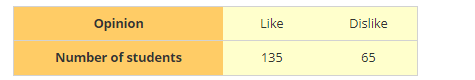# To know the opinion of the students about Mathematics, a survey of 200 students were conducted.

Question:

To know the opinion of the students about Mathematics, a survey of 200 students were conducted. The data was recorded in the following tableFind the probability that student chosen at random:

1. Likes Mathematics

2. Does not like it.

Solution:

1. Probability that a student likes mathematics

$=\frac{\text { Favorable out come }}{\text { Total out come }}$

= 135/200

= 0.675

2. Probability that a student does not like mathematics

$=\frac{\text { Favorable out come }}{\text { Total out come }}$

= 65/200

= 0.325